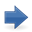Autonomous demand and economic growth: some empirical evidence

CSWP 13 (October 2015)

Author Daniele Girardi and Riccardo Pariboni

Keywords Growth, Effective Demand, Supermultiplier

JEL E11, E12, B51, O41

According to the Sraffian supermultiplier model, economic growth is driven by autonomous demand (exports, public spending and autonomous consumption). This paper tests empirically some major implications of the model. For this purpose, we calculate time-series of the autonomous components of aggregate demand and of the supermultiplier for the US, France, Germany, Italy and Spain and describe their patterns in recent decades. Changes in output and in autonomous demand are tightly correlated, both in the long and in the short-run. The supermultiplier is substantially higher and more stable in the US, while in the European countries it is lower and decreasing. Where the supermultiplier is reasonably stable - i.e., in the US since the 1960s - autonomous demand and output share a common long-run trend (i.e, they are cointegrated). The estimation of a Vector Error-Correction model (VECM) on US data suggests that autonomous demand exerts a long-run effect on GDP, but also that there is simultaneous causality between the two variables. We then estimate the multiplier of autonomous spending through a panel instrumental-variables approach, finding that a one dollar increase in autonomous demand raises output by 1.6 dollars over four years. A further implication of the model that we test against empirical evidence is that increases in autonomous demand growth tend to be followed by increases in the investment share. We find that this is the case in all five countries. An additional 1% increase in autonomous demand raises the investment share by 0.57 percentage points of GDP in the long-run.Download the paper